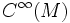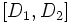# Lie bracket of differential operators

(diff) ← Older revision | Latest revision (diff) | Newer revision → (diff)
Let$M$ be a differential manifold and$D_1,D_2$ be differential operators from$C^\infty(M)$ to$C^\infty(M)$. The Lie bracket of$D_1$ and$D_2$ denoted$[D_1,D_2]$ is defined as the following differential operator:$D_1 \circ D_2 - D_2 \circ D_1$